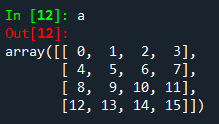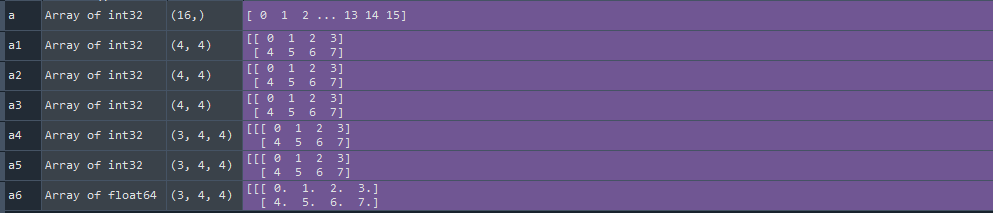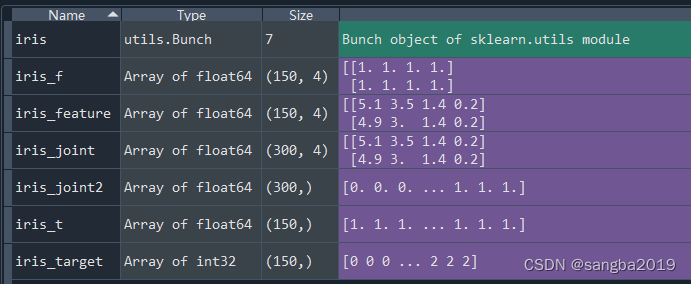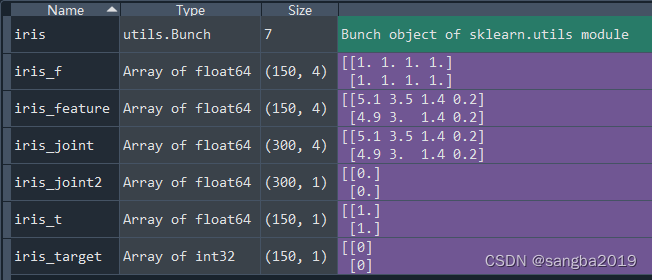• python两个二维数组合并
千次阅读
2022-04-02 17:21:20

如果多个二维数组维数一致, 那么可以直接相连, 再用np.array

a = np.array([[1,2,3],[4,5,6]])
b = np.array([[2,2,3],[4,5,6]])
c = np.array([[3,2,3],[4,5,6]])

com = np.array([a,b,c])


如果二维数组的维数不一致, 要曲线救国了. 先转化成list, 在变成array

aa = np.array([[[1,2,3],[4,5,6]],[[2,2,3],[4,5,6]],[[3,2,3],[4,5,6]]])
a = np.array([[4,2,3],[4,5,6]])

aa = aa.tolist(aa)
a = a.list(a)
aa.append(a)

com = np.array(aa)python
更多相关内容
• 假设a是一个4x4的数组： 想通过将两个a组合成[4,4,2]的形状得到数组b，可以通过如下方法： a = a[:,:,np.newaxis] b = np.concatenate((a,a),axis=2)

假设a是一个4x4的数组：想通过将两个a组合成[4,4,2]的形状得到数组b，可以通过如下方法：

a = a[:,:,np.newaxis] #新增维度
b = np.concatenate((a,a),axis=2) #合并

展开全文python
• 本文实例讲述了python实现合并两个数组的方法。分享给大家供大家参考。具体如下： python合并两个数组，将两个数组连接成一个数组，例如，数组 a=[1,2,3] ,数组 b=[4,5,6]，连接后：[1,2,3,4,5,6] 方法1 a=[1,2,3] ...
• 今天小编就为大家分享一篇Python numpy实现二维数组和一维数组拼接的方法，具有很好的参考价值，希望对大家有所帮助。一起跟随小编过来看看吧
• 问题: 存在多个独立的维度大小相同的二维数组（矩阵或者经纬度网格），将其合并为一个三维数组（矩阵），合并后的三维数组（矩阵）的某一维（如第一维）为...3、先构建一个空的三维数组，再将每一个二维数组填入 下面

## 问题:

• 存在多个独立的维度大小相同的二维数组（矩阵或者经纬度网格），将其合并为一个三维数组（矩阵），合并后的三维数组（矩阵）的某一维（如第一维）为矩阵的个数。

形象一点来举个例子

• 现在有三个4x4的二维数组a,b,c，想要将其合并为一个3x4x4的三维数组

方法：

• 1、通过np.array([a,b,c]),变相的达到拓展维度的结果
• 2、通过np.vstack()先按顺序垂直地(逐行)堆栈数组，然后reshape为（3，4，4）的三维数组
• 3、先构建一个空的三维数组，再将每一个二维数组填入

下面是代码举例：

### 导入numpy库
import numpy as  np
#prepare data
a = np.arange(16)
a1=a.reshape([4,4])
a2=a1
a3=a1
### way 1

a4=np.array([a1,a2,a3])

### way 2
a5=np.vstack((a1,a2,a3)).reshape(3,4,4)
### way 3
a6=np.zeros((3,4,4))
a6[0,:,:]=a1
a6[1,:,:]=a2
a6[2,:,:]=a3

• 运行结果如下，发现基本都能实现需求。基于上述方法，可以实现其他数据的相关处理，比如合并多个二维经纬度网格数据、其他气象数据等等展开全文python
• iris_target = iris.target #分类数据 iris_f = np.ones(iris_feature.shape) iris_t = np.ones(iris_target.shape) iris_target = iris_target.reshape(len(iris_target),1) #将一维数组转为二维数组 iris_t = iris...

import pandas as pd
import numpy as np
import matplotlib.pyplot as plt

from sklearn.datasets import load_iris

iris = load_iris()  #加载鸢尾花数据集
iris_feature = iris.data  #特征数据
iris_target = iris.target  #分类数据
iris_f = np.ones(iris_feature.shape)
iris_t = np.ones(iris_target.shape)

iris_joint = np.concatenate((iris_feature,iris_f),axis=0) #axis=0沿着纵轴拼接，axis=1沿着横轴拼接
iris_joint2 = np.concatenate((iris_target,iris_t),axis=0)


结果import pandas as pd
import numpy as np
import matplotlib.pyplot as plt

from sklearn.datasets import load_iris

iris = load_iris()  #加载鸢尾花数据集
iris_feature = iris.data  #特征数据
iris_target = iris.target  #分类数据
iris_f = np.ones(iris_feature.shape)
iris_t = np.ones(iris_target.shape)
iris_target = iris_target.reshape(len(iris_target),1) #将一维数组转为二维数组
iris_t = iris_t.reshape(len(iris_t),1)

iris_joint = np.concatenate((iris_feature,iris_f),axis=0) #axis=0沿着纵轴拼接，axis=1沿着横轴拼接
iris_joint2 = np.concatenate((iris_target,iris_t),axis=0)


结果展开全文python
• 今天小编就为大家分享一篇python实现两个一维列表合并成一个二维列表，具有很好的参考价值，希望对大家有所帮助。一起跟随小编过来看看吧
• 1. 使用 + a1=[1,1,1] a2=[2,2,2] a3 = a1 + a2 print(a3) # 1 1 1 2 2 2 2. extend a1=[1,1,1] a2=[2,2,2] ...# 先生成新的二维数组a3 a3 = [a1, a2] #列表推导形成新的数组 a4 = [ y for a in a3 f
• 采用numpy快速将两个矩阵或数组合并成一个数组： import numpy as np 数组 a = [[1,2,3],[4,5,6]] b = [[1,1,1],[2,2,2]] 1、数组纵向合并 1) c = np.vstack((a,b)) c = array([[1, 2, 3], [4, 5, 6], [1, 1, ...
• reshape(shape) ： 不改变数组元素，返回一shape形状的数组，原数组不变。是对每行元素进行处理resize(shape) ： 与.reshape()功能一致，但修改原数组In : a = np.arange(20)#原数组不变In : a.reshape([4,5...
• Python中创建二维数组二维数组二维数组本质上是以数组作为数组元素的数组，即“数组的数组”，类型说明符 数组名[常量表达式][常量表达式]。二维数组又称为矩阵，行列数相等的矩阵称为方阵。对称矩阵a[i][j] = a[j]...
• Python输入的程序归类 输入一个一维数组 ...2. 输入一个二维数组 # 输入一个二维数组 n = int(input()) line = [*n]*n for i in range(n): line[i] = input().split(" ") line[i] = [int(j)python
• reshape(shape) ： 不改变数组元素，返回一shape形状的数组，原数组不变。是对每行元素进行处理 resize(shape) ： 与.reshape()功能一致，但修改原数组 In : a = np.arange(20)#原数组不变In [2...
• 项目中希望把一RGB图和gray图进行合并，然后进行后续的数据处理，如下方式可实现图之间的合并 import cv2 import numpy as np from PIL import Image from torch.utils.data.dataset import Dataset h,w =...python
• reshape(shape) ： 不改变数组元素，返回一shape形状的数组，原数组不变。是对每行元素进行处理resize(shape) ： 与.reshape()功能一致，但修改原数组In : a = np.arange(20)#原数组不变In : a.reshape([4,5...
• 两个一维数组合成2维数组 1、使用zip函数 zip() 函数用于将可迭代的对象作为参数，将对象中对应的元素打包成一个个元组，然后返回由这些元组组成的对象，这样做的好处是节约了不少的内存。 我们可以使用 list() 转换...python
• 撰写时间：2017.5.23一...print a.shape输出的值应该为（3，）二维数组2.numpy初始化二维数组a = np.array([[1,2,3]]); b = np.array([,,]);print a.shape//(1，3） print b.shape//（3,1）注意（3，）和（3,python 二维数组
• 我有两个地理坐标列表(lat,long),我需要将它们组合成一个2D数组或矩阵.它们现在存储在数据帧中：lat lon0 48.010258 -6.1569091 48.021648 -6.1058872 48.033028 -6.0548013 48.044384 -6.0036914 48.055706 -5....
• 假设有a1和a2两个数组： a1=[1,2,3] a2=[4,5,6] 合并方式 1.直接相加 #合并后赋值给新数组a3 a3 = a1 + a2 2.extend #调用此方法，a1会扩展成a1和a2的内容 a1.extend(a2) 3.列表表达式 ''' 学习中遇到问题没人...python...